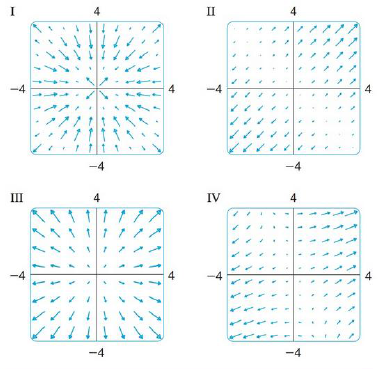Chapter 16.1, Problem 29E

Chapter
Section
Textbook Problem

Match the functions f with the plots of their gradient vector fields labeled I-IV. Give reasons for your choices.29. f(x, y) = x2 + y2To determine

To match: The function f(x,y)=x2+y2 with the plots labelled as I-IV.

Explanation

Given data:

f(x,y)=x2+y2

Formula used:

Write the expression for gradient vector field of two dimensional vector.

f(x,y)=fxi+fyj (1)

Write the required differentiation formulae with respect to x as follows.

x(x2)=2xx(y2)=0

Write the required differentiation formulae with respect to y as follows.

y(x2)=0y(y2)=2y

Differentiate the term x2+y2 with respect to x .

x(x2+y2)=x(x2)+x(y2)=2x+0=2x

Differentiate the term x2+y2 with respect to y

Still sussing out bartleby?

Check out a sample textbook solution.

See a sample solution

The Solution to Your Study Problems

Bartleby provides explanations to thousands of textbook problems written by our experts, many with advanced degrees!

Get Started

In Exercises 1728, use the logarithm identities to obtain the missing quantity.

Finite Mathematics and Applied Calculus (MindTap Course List)

In Exercises 7-28, perform the indicated operations and simplify each expression. 13. 2x2x13x2x+5

Applied Calculus for the Managerial, Life, and Social Sciences: A Brief Approach

Evaluate the limit, if it exists. limx4x2+95x+4

Single Variable Calculus: Early Transcendentals

True or False: converges conditionally.

Study Guide for Stewart's Single Variable Calculus: Early Transcendentals, 8th